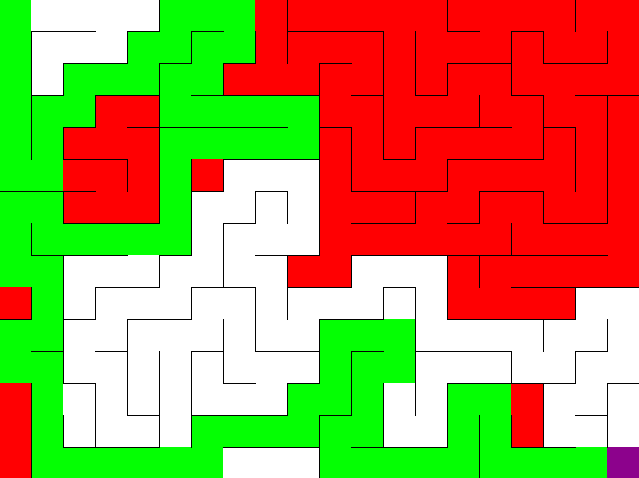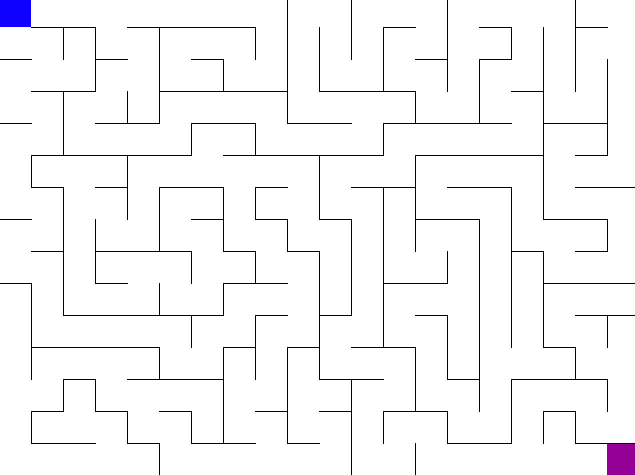# Searching a maze using DFS and BFS in Python 3

I solved the problem posted from Make School Trees and Maze article, which asks me to searching a maze using DFS and BFS in Python.

Here's the final output from PyGame:I would like to ask for code review, as I paste my code snippet below: Here's my DFS and BFS solution for solve_maze.py code review for implementation.

BFS solutino looks like this:You can find my code for generating_maze here: https://github.com/Jeffchiucp/graph-maze-problem

import maze
import generate_maze
import sys
import random

BIT_SOLUTION = 0b0000010010010110
# Solve maze using Pre-Order DFS algorithm, terminate with solution
def solve_dfs(m):
stack = []
current_cell = 0
visited_cells = 1

while current_cell != m.total_cells -1:
print(current_cell)
unvisited_neighbors = m.cell_neighbors(current_cell)
if len(unvisited_neighbors) >= 1:
# choose random neighbor to be new cell
new_cell_index = random.randint(0, len(unvisited_neighbors) - 1)
new_cell, compass_index = unvisited_neighbors[new_cell_index]
# knock down wall between it and current cell using visited_cell
m.visit_cell(current_cell, new_cell, compass_index)
# push current cell to stack
stack.append(current_cell)
# set current cell to new cell
current_cell = new_cell
# add 1 to visited cells
visited_cells += 1
else:
m.backtrack(current_cell)
current_cell = stack.pop()
print("run")
m.refresh_maze_view()
m.state = 'idle'

# Solve maze using BFS algorithm, terminate with solution
def solve_bfs(m):
"""
create a queue
set current cell to 0
set in direction to 0b0000
set visited cells to 0
enqueue (current cell, in direction)

while current cell not goal and queue not empty
dequeue to current cell, in direction
visit current cell with bfs_visit_cell
call refresh_maze_view to update visualization

get unvisited neighbors of current cell using cell_neighbors, add to queue

trace solution path and update cells with solution data using reconstruct_solution

set state to 'idle'
"""
queue = []
cur_cell = 0
in_direction = 0b0000
visited_cells = 0
queue.insert(0, (cur_cell, in_direction))
while not cur_cell == len(m.maze_array) - 1 and len(queue) > 0:
cur_cell, in_direction = queue.pop()
m.bfs_visit_cell(cur_cell, in_direction)
visited_cells += 1
m.refresh_maze_view()
neighbors = m.cell_neighbors(cur_cell)
for neighbor in neighbors:
queue.insert(0, neighbor)
m.reconstruct_solution(cur_cell)
m.state = "idle"

def print_solution_array(m):
solution = m.solution_array()
print('Solution ({} steps): {}'.format(len(solution), solution))

def main(solver='dfs'):
current_maze = maze.Maze('create')
generate_maze.create_dfs(current_maze)
if solver == 'dfs':
solve_dfs(current_maze)
elif solver == 'bfs':
solve_bfs(current_maze)
while 1:
maze.check_for_exit()
return

if __name__ == '__main__':
if len(sys.argv) > 1:
main(sys.argv)
else:
main()

• I thought it was a fun problem to solve – NinjaG Jul 2 '18 at 23:02

### Kill the noise

It's great that the solution works, but it's full of elements that seem to serve no purpose, which makes it confusing and hard to read.

• visited_cells is modified but never used
• BIT_SOLUTION is defined but never used
• A comment like # add 1 to visited cells adds no value to a code like visited_cells += 1. Avoid writing such comments.
• The same goes for the # Solve maze ... comments.
• The doc comment """ ... """ in solve_bfs is inappropriate. Instead of useful documentation, it's pseudo-code of the implementation. It's unnecessary.
• The return statement is unnecessary at the end of a function.
• Why write 0b0000 instead of simply 0?

### Confusion

I'm confused by the different terminating condition in the two implementations. In one of them, reaching the goal is expressed as cur_cell == len(m.maze_array) - 1, in the other it's current_cell == m.total_cells - 1. It's best when there's one clear way to do something. I suggest to change the maze implementation so that the terminating condition can be expressed as m.is_goal(cell_id).

### Encapsulation

The posted code is a client of the maze library (I guess your own). It knows too much about how the maze is implemented. It's not good that it accesses implementation details such as m.maze_array and m.total_cells. It's not good that the client knows that the last cell in the implementation is the exit of the maze. It's not good that the client knows that the cells of the maze are stored in a list (maze_array). This also limits the kind of mazes that can be modeled.

If you change the maze API so that clients can check if they have reached the exit by calling m.is_goal(cell_id), then the maze library will be free to place the exit to wherever it likes in its storage, it wouldn't need to be the last. The client also wouldn't know what data structure was used to store the cells of the maze, which again would give the maze library the freedom to use whatever it likes, and the freedom to change the underlying storage as needed, for example to something more efficient in a future release.

### Style

You can replace len(v) > 0 and len(v) >= 1 with simply v, because a non-empty collection is truthy in Python.

Instead of while 1:, it's more natural to write while True:.